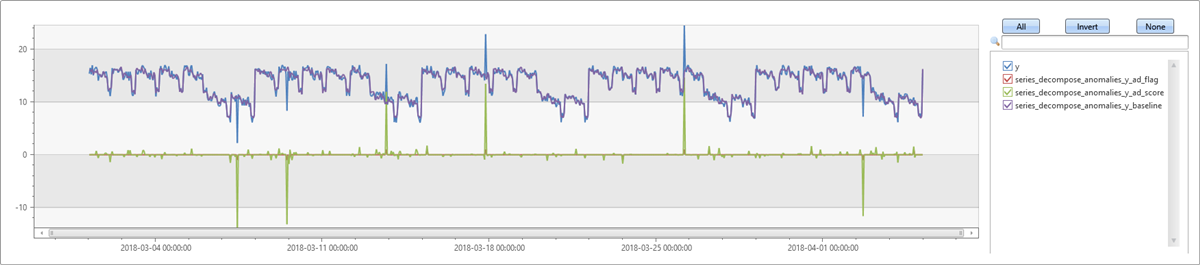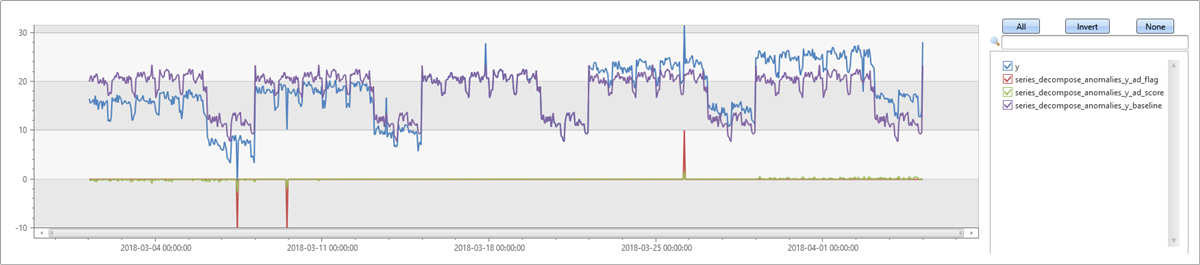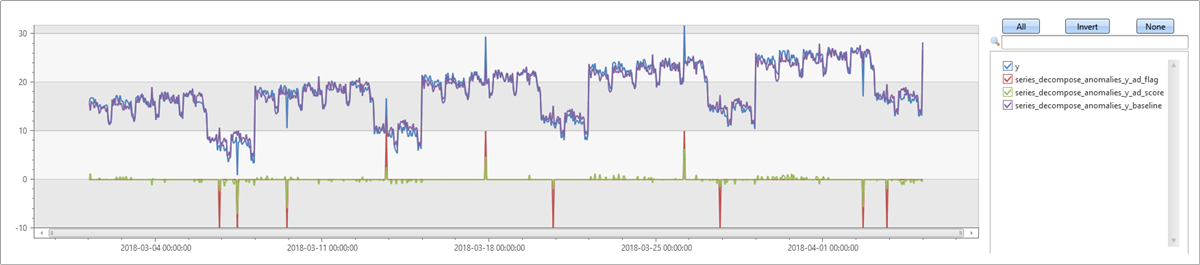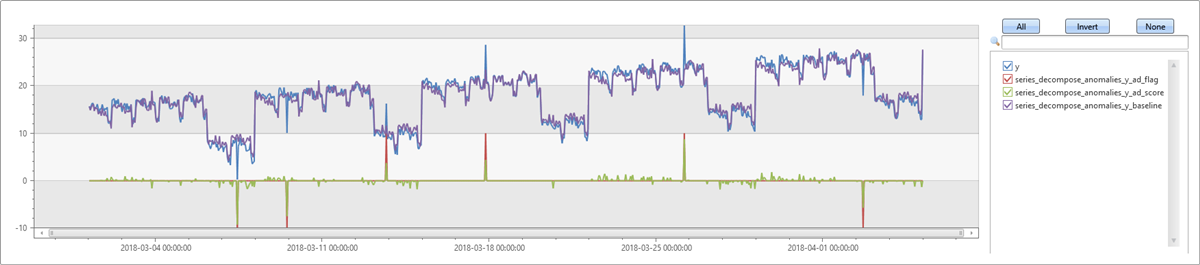# series_decompose_anomalies()

## 语法

`series_decompose_anomalies (`Series`[, `Threshold`,`Seasonality`,`Trend`, `Test_points`, `AD_method`,`Seasonality_threshold`])`

## 参数

• Series：动态数组单元格（数值数组），通常是 make-seriesmake_list 运算符生成的输出
• 阈值：异常阈值，默认值为 1.5（k 值），用于检测轻度异常或更严重的异常
• Seasonality：一个用于控制周期性分析的整数，其中包含
• -1：使用 series_periods_detect 自动检测周期性 [默认值]
• 0：无周期性（即，跳过提取此组件的操作）
• 期间：一个正整数，按箱单元数指定所需期间。 例如，如果将序列按 1 小时的箱分箱，则一个每周期间为 168 箱
• Trend：一个用于控制趋势分析的字符串，包含
• “avg”：将趋势组件定义为系列的平均值 [默认值]
• “none”：无趋势，跳过提取此组件的操作
• “linefit”：使用线性回归提取趋势组件
• Test_points：0 [默认值] 或正整数，指定要从学习（回归）过程中排除的序列末尾处的点的数目。 应当设置此参数，用于预测
• Seasonality_threshold：当 Seasonality 设置为 autodetect 时周期性分数的阈值。 默认分数阈值为 `0.6`。 有关详细信息，请参阅 series_periods_detect

## 返回

• `ad_flag`：一个三元序列，其中包含 (+1, -1, 0)，分别标记为正常/关闭/无异常
• `ad_score`：异常分数
• `baseline`：序列的预测值，通过分解获得

## 算法

1. 使用相应的参数调用 series_decompose() 来创建基线和残差序列。
2. 通过使用所选的异常情况检测方法对残差序列应用 series_outliers() 来计算 ad_score 序列。

## 示例

### 检测每周周期性中的异常

``````let ts=range t from 1 to 24*7*5 step 1
| extend Timestamp = datetime(2018-03-01 05:00) + 1h * t
| extend y = 2*rand() + iff((t/24)%7>=5, 10.0, 15.0) - (((t%24)/10)*((t%24)/10)) // generate a series with weekly seasonality
| extend y=iff(t==150 or t==200 or t==780, y-8.0, y) // add some dip outliers
| extend y=iff(t==300 or t==400 or t==600, y+8.0, y) // add some spike outliers
| summarize Timestamp=make_list(Timestamp, 10000),y=make_list(y, 10000);
ts
| extend series_decompose_anomalies(y)
| render timechart
``````### 检测具有趋势的每周周期性中的异常

``````let ts=range t from 1 to 24*7*5 step 1
| extend Timestamp = datetime(2018-03-01 05:00) + 1h * t
| extend y = 2*rand() + iff((t/24)%7>=5, 5.0, 15.0) - (((t%24)/10)*((t%24)/10)) + t/72.0 // generate a series with weekly seasonality and ongoing trend
| extend y=iff(t==150 or t==200 or t==780, y-8.0, y) // add some dip outliers
| extend y=iff(t==300 or t==400 or t==600, y+8.0, y) // add some spike outliers
| summarize Timestamp=make_list(Timestamp, 10000),y=make_list(y, 10000);
ts
| extend series_decompose_anomalies(y)
| extend series_decompose_anomalies_y_ad_flag =
series_multiply(10, series_decompose_anomalies_y_ad_flag) // multiply by 10 for visualization purposes
| render timechart
````````````let ts=range t from 1 to 24*7*5 step 1
| extend Timestamp = datetime(2018-03-01 05:00) + 1h * t
| extend y = 2*rand() + iff((t/24)%7>=5, 5.0, 15.0) - (((t%24)/10)*((t%24)/10)) + t/72.0 // generate a series with weekly seasonality and ongoing trend
| extend y=iff(t==150 or t==200 or t==780, y-8.0, y) // add some dip outliers
| extend y=iff(t==300 or t==400 or t==600, y+8.0, y) // add some spike outliers
| summarize Timestamp=make_list(Timestamp, 10000),y=make_list(y, 10000);
ts
| extend series_decompose_anomalies(y, 1.5, -1, 'linefit')
| extend series_decompose_anomalies_y_ad_flag =
series_multiply(10, series_decompose_anomalies_y_ad_flag) // multiply by 10 for visualization purposes
| render timechart
``````### 调整异常情况检测阈值

``````let ts=range t from 1 to 24*7*5 step 1
| extend Timestamp = datetime(2018-03-01 05:00) + 1h * t
| extend y = 2*rand() + iff((t/24)%7>=5, 5.0, 15.0) - (((t%24)/10)*((t%24)/10)) + t/72.0 // generate a series with weekly seasonality and onlgoing trend
| extend y=iff(t==150 or t==200 or t==780, y-8.0, y) // add some dip outliers
| extend y=iff(t==300 or t==400 or t==600, y+8.0, y) // add some spike outliers
| summarize Timestamp=make_list(Timestamp, 10000),y=make_list(y, 10000);
ts
| extend series_decompose_anomalies(y, 2.5, -1, 'linefit')
| extend series_decompose_anomalies_y_ad_flag =
series_multiply(10, series_decompose_anomalies_y_ad_flag) // multiply by 10 for visualization purposes
| render timechart
``````